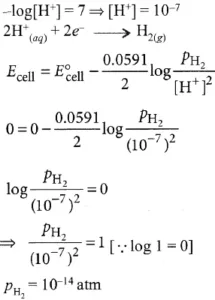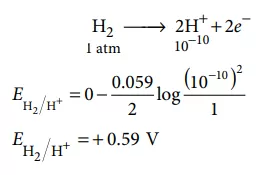# Electrochemistry NEET Important Solved QuestionsElectrochemistry Neet Important Solved Questions

Que 1. Which of the following statements is incorrect regarding electrochemistry?
(a) It is the study of the production of electricity from the energy released during spontaneous chemical reactions.
(b) NaOH, Cl2, alkali, and alkaline earth metals are prepared by electrochemical methods.
(c) The demerit associated with electrochemical methods is that they are more polluting. Thus they are ecodestructive.
(d) Electrochemical reactions are more energy-efficient and less polluting.
Ans 1. (c) Electrochemical reactions are more energy-efficient and less polluting. Thus the study of electrochemistry is important to create new eco-friendly technologies.

Que 2. For a spontaneous reaction the ΔG, equilibrium constant (K) and E°cell will be respectively:
(a) –ve, K > 1, +ve                                          (b) +ve, K > 1, –ve
(c) –ve, K < 1, –ve                                          (d) –ve, K > 1, –ve
Ans 2. (a) For spontaneous process, ΔG = –ve, K > 1 and E0Cell = +ve

Que 3. The standard electrode potential (E°) values of Al3+/Al, Ag+/Ag, K+/K and Cr3+/Cr are –1.66 V, 0.80 V, –2.93 V and –0.74 V, respectively. The correct decreasing order of reducing power of the metal is
(a) Ag > Cr > Al > K                                      (b) K > Al > Cr > Ag
(c) K > Al > Ag > Cr                                       (d) Al > K > Ag > Cr
Ans 3. (b)
Explanation: Higher the value of E° red, the stronger is the oxidising power. Thus, the decreasing order of reducing the power of the metal is K > Al > Cr > Ag.

Que 4. Which of the following statements about the galvanic cell is incorrect
(a) Anode is positive
(b) Oxidation occurs at the electrode with lower reduction potential
(c) Cathode is positive
(d) Reduction occurs at the cathode
Ans 4. a) Anode has a negative polarity

Electrochemistry Neet Important Solved Questions

Que 5. The electrical conductivity of the flowing aqueous solutions is highest for:
(a) 0.1 M CH3 COOH                                     (b) 0.1 M CH2 FCOOH
(c) 0.1 M CHF2 COOH                                   (d) 0.1 M CH2 ClCOOH
Ans 5. (c)
Explanation: Difluoroacetic acid will be the strongest acid, due to the electron-withdrawing effect of two fluorine atoms, so it will show maximum electrical conductivity.

Que 6. Standard reduction potentials of the half reactions are given below:
F2(g) + 2e  →  2F (aq); E° = + 2.85 V
Cl2(g)  + 2e2Cl– (aq); E° = + 1.36 V
Br2(l) + 2e → 2Br– (aq); E° = + 1.06 V
I2(s) + 2 e → 2I– (aq); E° = + 0.53 V
The strongest oxidising and reducing agents respectively are
(a) F2 and I–                                                   (b) Br2 and Cl–
(c)  Cl2 and Br–                                              (d) Cl2 and I2
Ans 6. (a)
Explanation: Less positive the value of reduction potential, stronger will be the reducing agent thus, I is the strongest reducing agent. More positive, the value of reduction potential shows good oxidising properties thus, the strongest oxidising agent is F2.

Que 7. Which device converts the chemical energy of a spontaneous redox reaction into electrical energy?
(a) Galvanic cell                                                         (b) Electrolytic cell
(c) Daniell cell                                                           (d) Both (a) and (c)
Ans 7. (d) Both (a) and (c)

Que 8. Conductivity (Seimens S) is directly proportional to the area of the vessel and the concentration of the solution in it and is inversely proportional to the length of the vessel, and then constant of proportionality is expressed in:
(a) S m mol–1                                                                (b) S2 m2 mol–2
(c) S m2 mol–1                                                               (d) S2 m2 mol
Ans 8. (c) S m2 mol–1
Explanation: S ∝ area (m2)
∝ Concentration (mol/m3)
Inversely proportional to Length

Electrochemistry Neet Important Solved Questions

Que 9. The pressure of H2 required to make the potential ofH2 electrode zero in pure water at 298 K is
(a) 10 -10 atm                                                              (b) 10 -4 atm
(c)  10–14 atm                                                              (d) 10–12  atm.
Ans 9. (c)
Explanation:Que 10. Standard electrode potential for Sn4+/Sn2+ couple is + 0.15 V and that for the Cr3+/Cr couple is – 0.74 V. These two couples in their standard state are connected to make a cell. The cell potential will be
(a) + 1.19 V                                                                (b) + 0.89 V
(c) + 0.18 V                                                                (d) + 1.83 V
Ans 10. (b)
Explanation: Given
E Sn4+/Sn2+ = + 0.15 V
E Cr3+/Cr = -0.74 V
ECell = ERed – Eoxi
= 0.74 + 0.15 = 0.89 V

Que 11. Electrolyte KCl, KNO3 , HCl, NaOAc, NaCl (Scm2 149.9 145 426.2 91.0 126.5 mol–1) Calculate using appropriate molar conductance of the electrolytes listed above at infinite dilution in H2O at 25°C:
(a) 517.2                                                                     (b) 552.7
(c) 390.7                                                                     (d) 217.5
Ans 11. (c)
Explanation: Λ (AcOH) = Λ (AcONa) + Λ (HCl) – Λ (NaCl)
= 91.0 + 426.2 – 126.5 = 390.7 S cm2 mol–1.

Que 12. A hydrogen gas electrode is made by dipping platinum wire in a solution of HCl of pH = 10 and by passing hydrogen gas around the platinum wire at one atm pressure. The oxidation potential of electrode would be
(a) 0.118 V                                                                 (b) 1.18 V
(c) 0.059 V                                                                 (d) 0.59 V
Ans 12. (d)
Explanation:Electrochemistry Neet Important Solved Questions

Que 13. Standard reduction potentials of the half-reactions are given below:
F2(g) + 2 e → 2 F (aq); E° = + 2.85 V
Cl2(g) + 2 e→ 2 Cl(aq); E° = + 1.36 V
Br2(l) + 2 e → 2 Br(aq); E° = + 1.06 V
I2(s) + 2 e → 2 I(aq); E° = + 0.53 V
The strongest oxidising and reducing agents respectively are
(a) F2 and I                                                                (b) Br2 and Cl
(c) Cl2 and Br                                                            (d) Cl2 and I2
Ans 13. (a)
Explanation: Higher the value of reduction potential higher will be the oxidising power whereas the lower the value of reduction potential higher will be the reducing power.

Que 14. The equivalent weight of Na2S2O3 in the reaction 2Na2S2O3 + I2 → Na2S4O6 + 2NaI is (M = molecular weight):
(a) M                                                                           (b) M/4
(c) M/0.5                                                                     (d) M/2
Ans 14. (a)
Explanation: As E = M/Change in oxidation number = M/1 = M

Que 15. A hydrogen gas electrode is made by dipping platinum wire in a solution of HCl of pH = 10 and bypassing hydrogen gas around the platinum wire at one atm pressure. The oxidation potential of the electrode would be
(a) 0.118 V                                                                 (b) 1.18 V
(c) 0.059 V                                                                 (d) 0.59 V
Ans 15. (d)
Explanation:Electrochemistry Neet Important Solved Questions